# Santaló formula

(diff) ← Older revision | Latest revision (diff) | Newer revision → (diff)

A formula describing the Liouville measure on the unit tangent bundle of a Riemannian manifold in terms of the geodesic flow and the measure of a codimension-one submanifold (see [a5] and [a6], Chap. 19).

Letbe an-dimensional Riemannian manifold, let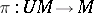be the unit tangent bundle of, letbe the Liouville measure on, and letbe the geodesic flow. One way to define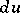is to start with the standard contact form(cf. Contact structure) and define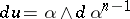. Liouville's theorem says thatis invariant under the geodesic flow(sinceis). Locally,is just the product measurewhereis the Riemannian volume form and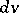is the standard volume form on the unit-sphere.

For any (locally defined) codimension-one submanifold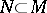, letbe the Riemannian volume element of the submanifold. Let, and, for each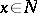, letbe a unit normal toat. Then there is a smooth mapping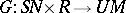, given by. Santaló's formula says: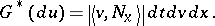The formula is used to convert integrals over subsetsof the unit tangent bundle to iterated integrals, first over a fixed unit-speed geodesic (say parametrized on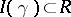) and then over the spaceof geodesics which are parametrized by their intersections with a fixed codimension-one submanifold and endowed with the measure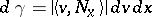, i.e.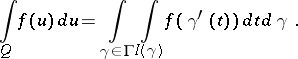One of the most important applications is to the study of Riemannian manifolds with smooth boundary. In this case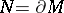,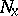is the inwardly pointing unit normal vector and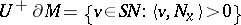. For any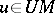, set. Note thatmeans that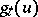is defined for all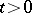. Let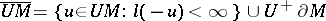, i.e.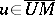meansfor some someand some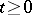. In this setting, Santaló's formula takes the form: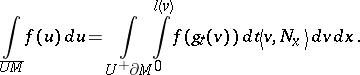One immediate application, by simply putting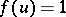, is: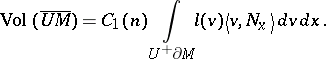Since the Liouville measure is locally a product measure, in the special casethis says.

The formula is often used to prove isoperimetric and rigidity results. A sample of such applications can be found in the references. See [a1] for Santaló's formula for time-like geodesic flow on Lorentzian surfaces.

How to Cite This Entry:
Santaló formula. Encyclopedia of Mathematics. URL: http://encyclopediaofmath.org/index.php?title=Santal%C3%B3_formula&oldid=18408
This article was adapted from an original article by C. Croke (originator), which appeared in Encyclopedia of Mathematics - ISBN 1402006098. See original article